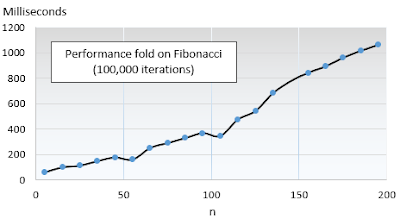## Thursday, July 9, 2015

### Fibonacci Recursive Implementation Galore

This post evaluates the performance of the tail recursion in Scala relative to alternative solutions as applied to the Fibonacci formula

Overview
There are many ways to skin a cat and implement the Fibonacci recursion. This post illustrates the power of tail recursion in Scala, relative to alternative solution such as recursion without tail elimination and iterations. This post compares the performance of the simple "out-of-the-box" implementation of the Fibonacci recursion with an Scala implementation using tail-recursion.

Performance of non-tail recursive Fibonacci
The Fibonacci algorithm is defined as
   f(n) = f(n-1) + f(n-2)
f(0) = 0
f(1) = 1

Let's look at text book recursive implementation.

def fibonacci(n: Int): Long =  {
if(n == 0) 0
else if(n == 1) 1
else fibonacci1(n-1) + fibonacci1(n-2)
}


The duration of execution is recorded for values between 2 and 50, run 10,000 times.The profile of the performance of the non-tail recursion of Fibonacci should not be a surprise. After all, the time complexity of this implementation is a staggering O(2n)

Tail-recursive implementation
Let's look at one example of implementation of the Fibonacci formula using the Scala tail recursion.

def fibonacci(n: Int): Long = {
@tailrec
def _fibonacci(i: Int, next: Long, sum: Long): Long = {
if(i ==0) sum
else fibonacci(i-1, sum+ next, next)
}
fibonacci(n, 1, 0)
}


The performance of the tail recursion is measure against a plain-vanilla iterative execution. The duration of execution is recorded for values between 5 and 200, run 100,000 times.

def fibonacci(n: Int): Long = {
var s1 = 0
var s2 = 1
(n-1 to 0 by -1).foreach(_ => {
val tmp = s2 + s1
s1 = s2
s2 = tmp
})
s1
}

br>
The following chart illustrates that the performance of the implementation of Fibonacci using the tail recursion and the iterative execution is almost identical.Implementation of iterative computation
For good measure, I thought it would be interesting to measure the performance of an iterative approach using a fold.

def fibonacci(n: Int): Long =
(n-1 to 0 by -1)./:((0, 1)){ case ((s1, s2), n) => {
val tmp = s2 + s1
(s2, tmp)
}}._1


Here is the performance profile for the iterative computation.Note: The fold (:/) can be implemented as a closure using a recursive formula for the function argument.

Wrapping up
The Scala tail recursion is indeed as effective as the iterative implementation at processing the Fibonacci formula. The performance of the recursion without tail elimination is extremely poor. Aggregators or reducers such as fold, reduce and scan add some overhead to the computation although the performance is still decent.

Reference

#### 1 comment:

1.The development of artificial intelligence (AI) has propelled more programming architects, information scientists, and different experts to investigate the plausibility of a vocation in machine learning. Notwithstanding, a few newcomers will in general spotlight a lot on hypothesis and insufficient on commonsense application. machine learning projects for final year In case you will succeed, you have to begin building machine learning projects in the near future.

Projects assist you with improving your applied ML skills rapidly while allowing you to investigate an intriguing point. Furthermore, you can include projects into your portfolio, making it simpler to get a vocation, discover cool profession openings, and Final Year Project Centers in Chennai even arrange a more significant compensation.

Data analytics is the study of dissecting crude data so as to make decisions about that data. Data analytics advances and procedures are generally utilized in business ventures to empower associations to settle on progressively Python Training in Chennai educated business choices. In the present worldwide commercial center, it isn't sufficient to assemble data and do the math; you should realize how to apply that data to genuine situations such that will affect conduct. In the program you will initially gain proficiency with the specialized skills, including R and Python dialects most usually utilized in data analytics programming and usage; Python Training in Chennai at that point center around the commonsense application, in view of genuine business issues in a scope of industry segments, for example, wellbeing, promoting and account.

The Nodejs Training Angular Training covers a wide range of topics including Components, Angular Directives, Angular Services, Pipes, security fundamentals, Routing, and Angular programmability. The new Angular TRaining will lay the foundation you need to specialise in Single Page Application developer. Angular Training